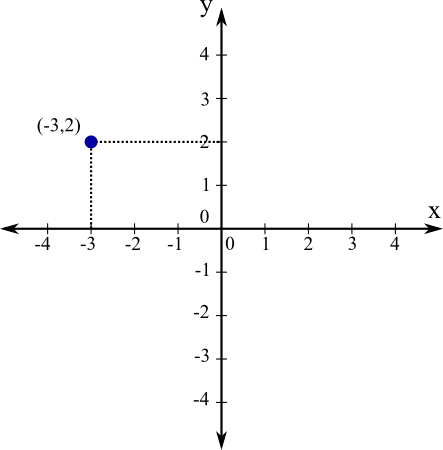# Math Insight

### Image: Cartesian axes in plane the plane with pointThe Cartesian coordinate system in the plane is illustrated by a point and the Cartesian axes. The point's coordinates are $(-3,2)$, as it is 3 units to the left and 2 units up from the origin, which is the intersection of the $x$- and $y$- axes.

Image file: cartesian_axes_2d_point.png

Source image file: cartesian_axes_2d_point.svg
Source image type: Inkscape SVG